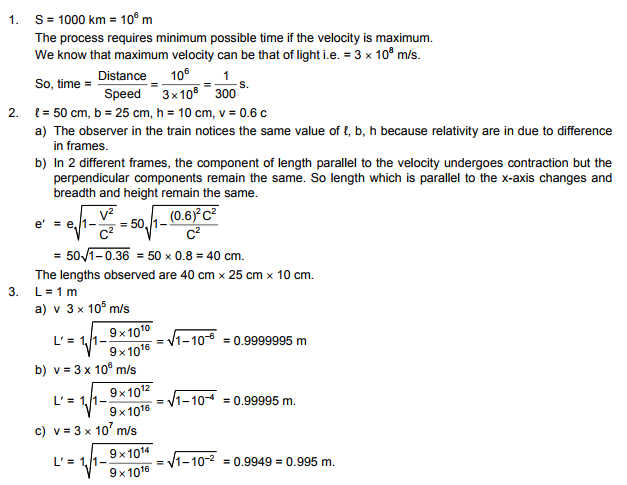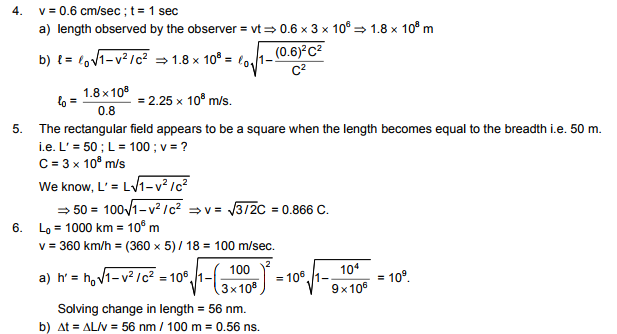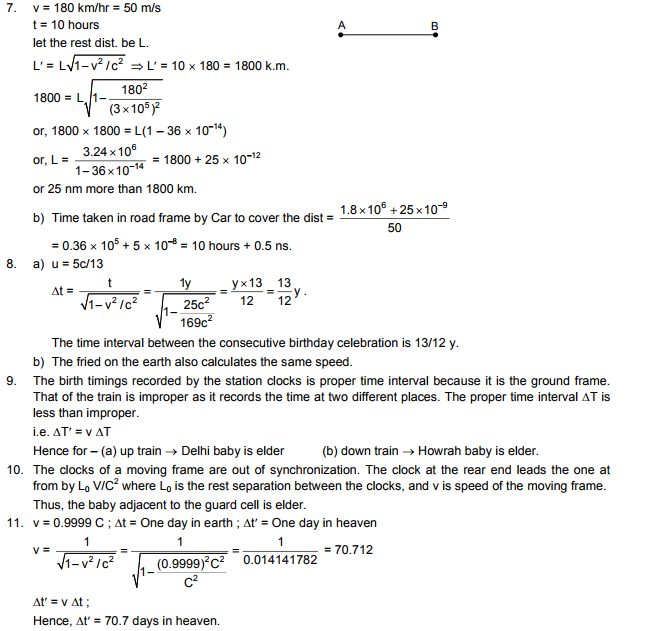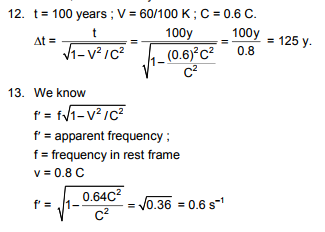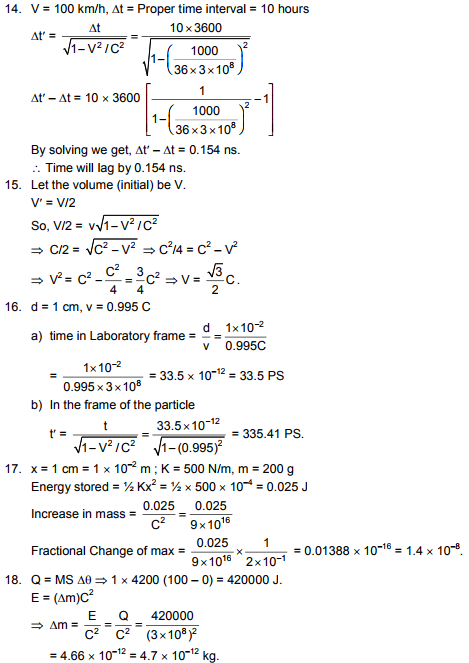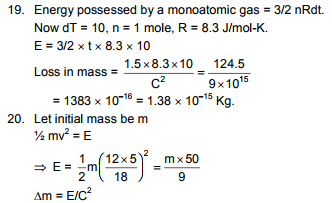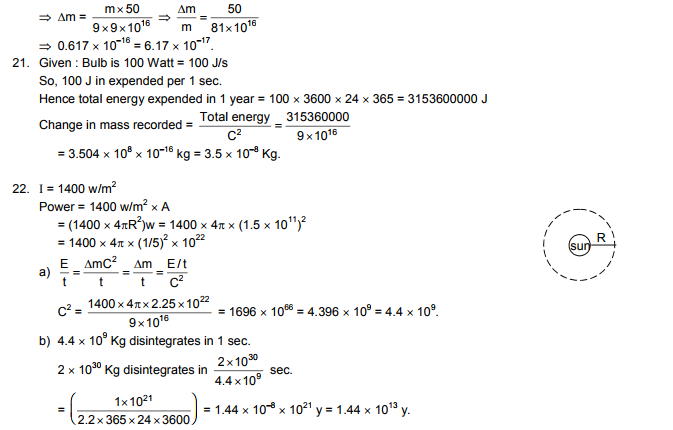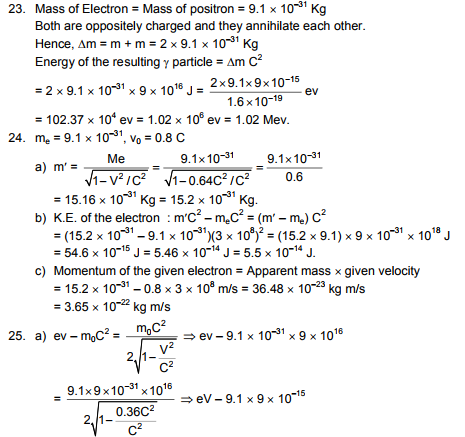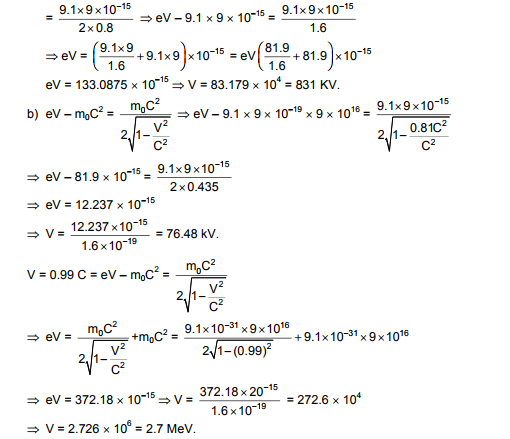# HC Verma Solutions Vol 2 Chapter 25 The Special Theory of Relativity

HC Verma Solutions Vol 2 Chapter 25 The Special Theory Of Relativity has been provided here to help students have a smooth preparation for their exams. The solution contains detailed and accurate answers to all the exercises and is key to understand the chapter and the concepts discussed in a much better way. The solution further ensures easy learning and enhances a student’s ability to solve even the most complex questions such as;

• Questions on finding the change in mass, power, total loss and gain in energy in particles.
• Questions related to the frictional change of mass, the energy stored and increase in the mass of particles.
• Questions based on time in the laboratory frame, frequency and speed of frames, etc.

The HC Verma solutions have been prepared by our experts with the main goal of giving students clear knowledge of the topics. The solutions will also be helpful for students who are preparing for competitive exams like JEE. Students can access the solutions PDF below.

## Key Topics Related To Special Theory of Relativity

Some of the important topics covered in this chapter are;

• The Principle of Relativity
• Kinematical Consequences
• Dynamics at Large Velocity
• Energy and Momentum
• The Ultimate Speed

## Important Questions In Chapter 25

1. It is given that the mass of a particle depends on its speed. On the basis of this statement, does the attraction of the earth on the particle depend on the particle’s speed also?

2. What will happen to a charged particle when it is projected at a very high-speed perpendicular to a uniform magnetic field?

3. When the speed of a particle is increased what changes will occur to its rest mass?

(a) changes (b) remains the same (c) increases (d) decreases

4. While measuring the length of a rod all motions are parallel to the length of the rod. Can you point out in which following cases the measured length will be minimum?

(a) The experimenter and the rod move with the same speed v in the same direction.

(b) The experimenter and the rod move with the same speed v in opposite directions.

(c) The experimenter stays at rest but the rod moves at speed v.

(d) The experimenter moves with the speed v but the rod stays at rest.

5. What will happen to the linear momentum if the speed of a particle moving at relativistic speed is doubled?

(a) will become double (b) more than double (c) it will remain equal (d) less than double

## HC Verma Solutions Vol 2 The Special Theory of Relativity Chapter 25